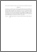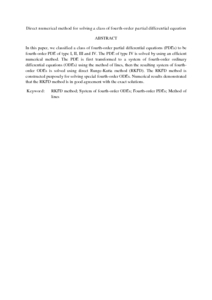# Direct numerical method for solving a class of fourth-order partial differential equation

## Citation

Hussain, Kasim Abbas and Ismail, Fudziah and Senu, Norazak (2016) Direct numerical method for solving a class of fourth-order partial differential equation. Global Journal of Pure and Applied Mathematics, 12 (2). pp. 1257-1272. ISSN 0973-1768; ESSN: 0973-9750

## Abstract

In this paper, we classified a class of fourth-order partial differential equations (PDEs) to be fourth-order PDE of type I, II, III and IV. The PDE of type IV is solved by using an efficient numerical method. The PDE is first transformed to a system of fourth-order ordinary differential equations (ODEs) using the method of lines, then the resulting system of fourth-order ODEs is solved using direct Runge-Kutta method (RKFD). The RKFD method is constructed purposely for solving special fourth-order ODEs. Numerical results demonstrated that the RKFD method is in good agreement with the exact solutions.Preview
PDF (Abstract)
Direct numerical method for solving a class of fourth-order partial differential equation.pdfView Item